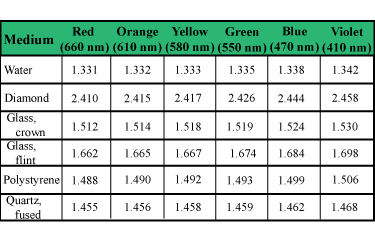# Problem: (a) A narrow beam of light containing yellow (580 nm) and green (550 nm) wavelengths goes from polystyrene to air, striking the surface at a 30.0° incident angle. What is the angle between the colors when they emerge? (b) How far would they have to travel to be separated by 1.00 mm?

###### FREE Expert Solution

Snell's law:

$\overline{){{\mathbf{\eta }}}_{{\mathbf{1}}}{\mathbf{s}}{\mathbf{i}}{\mathbf{n}}{{\mathbf{\theta }}}_{{\mathbf{1}}}{\mathbf{=}}{{\mathbf{\eta }}}_{{\mathbf{2}}}{\mathbf{s}}{\mathbf{i}}{\mathbf{n}}{{\mathbf{\theta }}}_{{\mathbf{2}}}}$

(a)

We are asked to determine the difference in θ2 for the two wavelengths.

Δθ = θ2g - θ2gy

θ2 = sin-1 ((η1sinθ1)/η2

80% (108 ratings)###### Problem Details(a) A narrow beam of light containing yellow (580 nm) and green (550 nm) wavelengths goes from polystyrene to air, striking the surface at a 30.0° incident angle. What is the angle between the colors when they emerge? (b) How far would they have to travel to be separated by 1.00 mm?